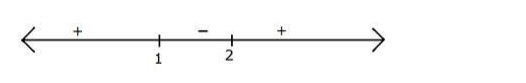# Find the solution set of the in equation

Question:

Find the solution set of the in equation $|2 x-3|<1$.

Solution:

$|2 x-3|<1$

Square both sides

$\Rightarrow(2 x-3)^{2}<1^{2}$

$\Rightarrow 4 x^{2}-12 x+9<1$

$\Rightarrow 4 x^{2}-12 x+8<0$

Divide throughout by 4

$\Rightarrow x^{2}-3 x+2<0$

$\Rightarrow x^{2}-2 x-x+2<0$

$\Rightarrow x(x-2)-1(x-2)<0$

$\Rightarrow(x-1)(x-2)<0$

Observe that when $x$ is greater than $2(x-1)(x-2)$ is positive

And for each root the sign changes henceWe want less than 0 that is negative part

Hence $x$ should be between 1 and 2 for $(x-1)(x-2)$ to be negative

Hence $x \in(1,2)$

Hence the solution set of $|2 x-3|<1$ is $(1,2)$